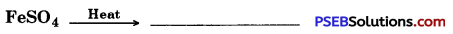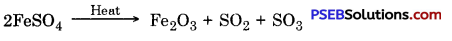# PSEB 10th Class Science Important Questions Chapter 1 Chemical Reactions and Equations

Punjab State Board PSEB 10th Class Science Important Questions Chapter 1 Chemical Reactions and Equations Important Questions and Answers.

## PSEB 10th Class Science Important Questions Chapter 1 Chemical Reactions and Equations

Question 1.
How do we write balanced chemical equations?
To understand the method to write balanced chemical equation, let us take an example.
Zinc + Sulphuric acid → Zinc sulphate + hydrogen This equation can be written as below
Zn + H2SO4 → ZnSO4 + H2

Check the number of atoms on both sides of the arrow.

 Element No. of atoms in reactants (LHS) No. of atoms in products (RHS) Zn 1 1 H 2 2 S 1 1 0 4 4

In the equation, number of atoms are same on the both sides of the arrow, thus it is balanced chemical equation.
Now let us try to balance the following chemical equation
Fe + H2O → Fe3O4 + H2

Step I: Make a list of atoms of different elements in the unbalanced equation

 Element No. of atoms in reactants (LHS) No. of atoms in products (RHS) Fe 1 3 H 2 2 0 1 4

Step II: Choose a compound with maximum number of atoms for balancing whether it is reactant or product. Choose an element with maximum number of atoms in the choosen compound. On this basis we choose Fe3O4 and in it we choose the element oxygen. On the right, there are four atoms of oxygen and on the left only one.

To balance, oxygen atom

 Atoms of oxygen In reactants In product (i) initially 1 (In H2O) 4 (In Fe3O4) (iii) after balancing 1 × 4 4

To equalize the number of atoms, we can not change the formulae of elements and compounds taking part in the reaction e.g. to balance oxygen atoms we can put coefficient ‘4’ and can write 4H20 but we cannot write H204 or (H2O)4 or (H2O4).

A partially balanced equation will be
Fe + 4H2O → Fe3O4 + H2
(Partially balanced equation)

Step III: Fe and H are still unbalanced. Choose any one and move ahead. To balance hydrogen atoms, on left side, make number at atoms of hydrogen ‘4’.

 Atoms of hydrogen In reactants In product Initially 8 (4 In H2O) 2 (In H2) After balancing 8 2 × 4

Now equation becomes
Fe + 4 H2O → Fe3O4 + 4H2

Step IV: Check the above equation and choose third element which still is unbalanced. We will find that only iron is the element, which remains to be balanced.

To balance Fe we take 3 atoms of Fe on the left side.
3Fe + 4 H2O → Fe3O4 + 4H2

Step V: At the end, let us count the number ofatoms of elements on both sides of the equation
3 Fe + 4H2O → Fe3O4 + 4H2 (Balanced equation)

Number of atoms of every element on both sides of the equation are equal. Therefore this equation is now balanced. This method of balancing chemical equations is known as hit and trial method because we use smallest integral coefficient to balance the equation.

Step VI: To write the symbols for physical states. There is no information given about the physical state in the above balanced equation.
To make chemical equation more informative, write the physical states with the reactants and products. To represent the states of solid, liquid, gas, aqueous of the reactants and products we write (s), (l), (g) and (aq) respectively with them. When reactant or product is present in the form of solution in water then word (aq) is written.

Thus, balanced equation will be
3Fe(s) + 4H2O (g) → Fe3O4(s) + 4H2(g)
when (g) is used with water, then it means that water is used in the form of steam in the reaction. Usually, physical state is not mentioned in the chemical equation until it is very necessary.

Sometimes the conditions of the reaction like temperature, pressure, catalyst etc. are shown over or under the arrow e.g.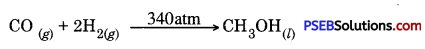Question 2.
Give types of chemical reactions with examples.
The various types of chemical reactions are :
1. Combination reactions: In these reactions two or more than two reactants combine to form a single product. Such reactions are known as combination reactions.

Example: Calcium oxide reacts with water to form calcium hydoxide (slaked lime) and a lot of heat is evolved.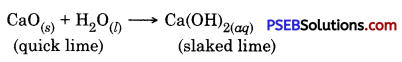In this reaction calcium oxide and water combine to give single product calcium hydroxide.

2. Dissociation or Decomposition reactions: In these reactions single reactant breaks into two or more than two new substances. Such reactions are called dissociation reactions.

Examples of dissociation reaction :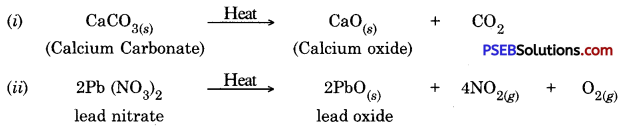3. Displacement reactions: When an element displaces another element from a compound, then it is called displacement reaction.

Examples: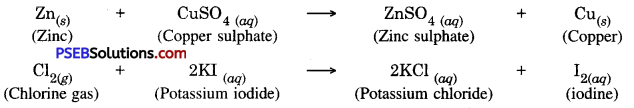4. Double displacement reactions. In such a reaction two different atoms or group of atoms exchange themselves with each other.

Example :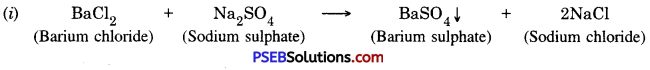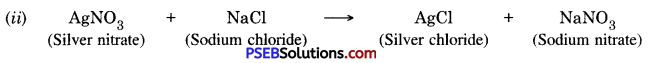Question 3.
Briefly describe oxidation and reduction with suitable examples.
Oxidation. Oxidation of a substance takes place in a reaction when there is gain of oxygen or loss of hydrogen in it and opposite to it reduction takes places when there is loss of oxygen or gain of hydrogen.

Example: A black coating of copper (II) oxide is seen on copper powder. Why this black substance is formed?
This copper oxide is formed due to combination of copper with oxygen.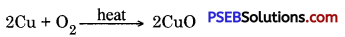If on this heated substance hydrogen gas is allowed to flow, this black coloured coating changes into brown colour because in these circumstances reverse reaction takes place and copper is again obtained.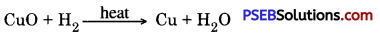When there is gain of oxygen in a substance in a reaction we say oxidation of the substance has taken place and when there is loss of oxygen we say reduction of the substance has taken place.

There is loss of oxygen in copper (II) oxide, therefore it gets reduced. There is gain of oxygen in hydrogen, therefore it is oxidized. Thus, in a reaction one of the reactant is oxidized and other reactant is reduced. Such type of reactions are called reduction-oxidation reactions or redox reactions.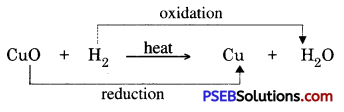Other examples of redox reaction are :

• ZnO + C → Zn + CO
• MnO2 + 4HCl → MnCl2 + 2H2O + Cl2
• In this reaction carbon is oxidized to become CO and ZnO is reduced to Zn.
• In this reaction HCl is oxidized to Cl2 and MnO2 is reduced to MnCl2.

Question 4.
Balance the following chemical equations.
(i) H2 + N2 → NH3
3H2 + N2 → 2NHg

(ii) BaCl2 + Al2(SO4)3 → AlCl3 + BaSO4
3BaCl2 + Al2(SO4)2 → 2AlCl3 + 3BaSO4

(iii) H2S + O2 → SO2 + H2O
2H2S + 3O2 → 2SO2 + 2H2O

(iv) KBr + BaI2 → KI +BaBr2
2KBr + BaI2 → 2KI + BaBr2

(v) Al + CuCl2 → AlCl3 + Cu
2Al + 3CuCl2 → 2AlCl3 + 3Cu

(vi) AgNOg + Cu → Cu(NO3)2 +Ag
2AgNOg + Cu → Cu(NO3)2 + 2Ag

(vii) Al (OH)3 → Al2O3 + H2O
2Al(OH)3 → Al2O3 + 3H2O

(viii) NH3 + CuO → Cu + N2 + H2O
2NH3 + 3CuO → Cu + N2 + 3H2O

(ix) KClO3 → KCl + O2
2KClO3 → 2KCl + 3O2

(x) BaCl2 + K2SO4 → 2BaSO4 + KCl
BaCl2 + K2SO4 → BaSO4 + 2KCl.

Question 1.
Give two examples from our daily life to show that chemical changes do take place in our daily life.

1. Fermentation of grapes take place.
2. Our body digests the food.
3. In summer, if milk is left in the room, we can see a change in it as it becomes sour.

Question 2.
Differentiate between balanced and skeletal chemical equation.
Balanced equation. If number of atoms of each element are same before and after the reaction, then such a reaction is balanced chemical reaction.

Skeletal chemical equation. When number of atoms of each element before and after the reaction are not same then such a chemical equation is called skeletal Chemical equation.

Question 3.
What are the necessary requirements while writing a chemical equation?
These are :

• It represents a true chemical change.
• It should be balanced.
• It should represent physical states of the reactants and products.
• It should indicate the necessary conditions required for the reaction to occur.
• It should represent heat changes during reactions.

Question 4.
Give one example each of balanced and skeletal equation.
2KCl + O2 → 2 KCl(s) + 3O2(g)
This is example of balanced equation.
Zn + AgNO3(aq) → Zn (NO3)2 + Ag
This is example of skeletal equation.

Question 5.
Give chemical formula for slaked lime and one use of it.
Chemical formula -Ca(OH)2. Solution of slaked lime is used for white washing the buildings.

Question 6.
Why there is a shine on the walls after two-three days of white washing?
Calcium hydroxide reacts slowly with carbon dioxide present in the air and form a thin layer of calcium carbonate on the walls. After 2-3 days of white wash, calcium carbonate is formed due to which there is a shine on the walls.

Question 7.
Give chemical formula of marble and give reaction to show bow it is formed?
Marble is also known as calcium carbonate.
Its chemical formula is CaCO3 Reaction to show its formation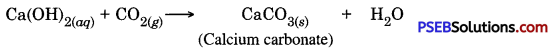Question 8.
MnO2 + 4HCl → MnCl2 + 2H2O + Cl2
How oxidation and reduction in the above reaction is taking place? What is the name of such reaction?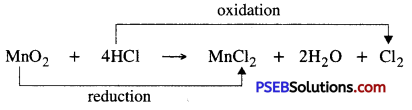Such a reaction is called redox reaction.

Question 9.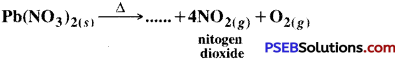Complete the above reaction and tell the colour of the product.
Above reaction is :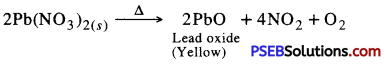colour of PbO is yellow.

Question 10.
Give colours of following solutions.
1. FeSO4
FeSO4 → green

2. CuS04
CuSO4 → blue

Question 11.
What is rusting? What is the chemical formula of rust. What is its loss?
New articles made up of iron are shiny but after some time a reddish brown coating is seen on their surface. Usually it is called rusting of iron.

Chemical formula of rust – Fe2O3.H2O (Hydrated iron (III) oxide)
It is powdery substance that gets removed from the surface and due to this iron articles are getting damaged.

Question 12.
Fats are oils containing foods, when kept for long time then what change take place in them? What is name of this process?
Fats and oils containing foods, when kept for long time, results in the change of their taste and smell. This process is named as rancidity.

Question 13.
How can we stop rancidity?

• To slower down the rate of oxidation, antioxidants are added to food items.
• By keeping food items in air tight containers.
• By flushing nitrogen gas in packets of chips so as to stop the oxidation of chips.

Question 14.
Zinc metal can displace copper from copper sulphate solution. But copper metal can not displace zinc from the solution of Zinc sulphate. Give reason.
zinc is more reactive than copper. It can displace copper from copper sulphate solution. Copper is less reactive than zinc thus copper can not displace zinc from zinc sulphate solution.

Question 15.
What happens when zinc rod is placed in copper sulphate solution? Give chemical equation for the reaction.
zinc is more reactive than copper. It wall displace copper from solution of copper sulphate and zinc sulphate is formed. Blue colour of the solution of copper sulphate change to white slowly.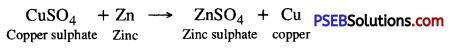Question 16.
What is lime mitigation? Why there is sound of shuun-shuun in this process? Give chemical equation representing the reaction.
When quick lime is mixed with water, it changes to slaked lime. This is lime mitigation. This is a exothermic reaction and heat is evolved due to which there is sound of shuun-shuun is produced.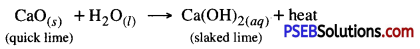Question 17.
Write names of the substances which got reduced and oxidized.
1. SO2 + 3H2S → 2H3O + 3S
In SO2, S is reduced and in H2S, S is oxidized.

2. 2Al + 3HCl → 2AlCl2 + 3H2
Aluminium is reduced and chlorine is oxidized.

3. 2H2S + SO2 → 3S + 2H2O
Hydrogen is oxidised and sulphur is reduced.

4. Zn + 2AgNOa → An (NO3)2 + 3Ag
Zinc is oxidised and silver is reduced.

5. H2 + CuO → Cu + H2O.
Hydrogen is oxidised and copper is reduced.

Question 18.
What does a complete chemical equation represent and why is it necessary to balance a chemical equation?
A complete chemical equation represents reactants, products and their physical state in a symbolic form.
A chemical equation is balanced so that number of atoms of every element in reactants and products becomes equal. Therefore it is necessary to balance the chemical equation.

Question 19.
What type of reactions are combustion of coal and formation of water? Represent by chemical equation.
1. Combustion of Coal:
C(s) + O2 (g) → CO2 (g)

2. Formation of water from H2 and O2 :
2H2(g) + O2(g) → 2H2O(l)

Question 20.
How is dissociation reaction different from combination reaction?
In a combination reaction two or more than two substance combine to give a single new substance where as in dissociation reaction a single substance breaks or dissociates into two or more than two new substances.

Question 21.
Give two examples of dissociation reaction.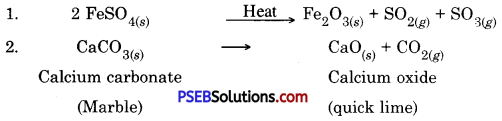Question 22.
What is the difference between oxidation and reduction?
In reaction, some substance gain or loose oxygen and hydrogen. If there is gain of oxygen or loss of hydrogen from a substance then it is called oxidation. Loss of oxygen and gain of hydrogen is called reduction.

Question 23.
Give two examples of corrosion.
Examples of corrosion are :

1. black coating on silver
2. green coating on copper.

Question 24.
Which type of reaction is shown in figure given below and define it.
Displacement Reaction. It is a reaction in which more active element (metal or non-metal) displaces less active element (metal or non-metal) from its solution of its compound.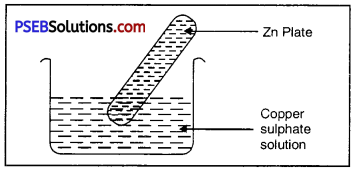Question 25.
What is shown in the figure given ahead? Also indicate 1 and 2 in the figure .
The figure represents electro-lysis of water :

1. Oxygen gas
2. Hydrogen gas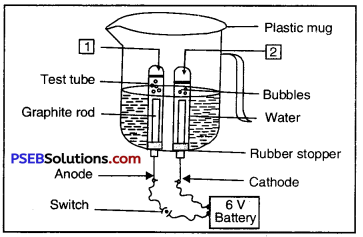Question 26.
The following diagram displays a chemical reaction. Observe carefully and answer the following questions: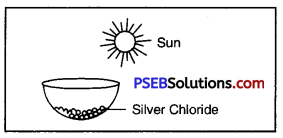(i) Identify the type of chemical reaction.
Photochemical decomposition

(ii) Write the chemical equations.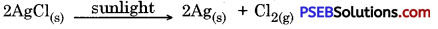Question 1.
What do we get on the combustion of magnesium ribbon?
We get a white powder of Magnesium oxide.

Question 2.
What is law of conservation of mass?
In any chemical reaction, mass can neither be created nor destroyed.

Question 3.
Define balanced chemical equation.
If number of atoms of each element before and after the chemical reaction are equal, then such a chemical reaction is balanced equation.

Question 4.
Give one example of balanced equation.
3 Fe + 4H2O → Fe3O4 + 4H2.

Question 5.
If (g) is written with water, then what does it represent?
Water is in the form of vapour or steam.

Question 6.
Sometimes we show the conditions of a reaction like pressure, temperature, catalyst etc. where do we write it.
Above and below the arrow.

Question 7.
How does a new substance form in a chemical reaction?
By breaking and making of bonds between atoms.

Question 8.
Define combination reaction.
A reaction in which two or more than two reactants combine to give single product is called combination reaction.

Question 9.
What is the use of slaked lime?
For whitewashing the buildings.

Question 10.
Write chemical formula for Marble.
CaCO3.

Question 11.
What do we get on the combustion of natural gas?
CO2, H2O and energy.

Question 12.
Write formula for ferrous sulphate.
FeSO4.7H2O.

Question 13.
What is formed of grey colour from silver chloride in sun light?
Silver metal.

Question 14.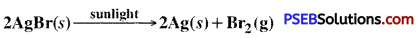Where is above reaction used?
Black and white photography.

Question 15.
Why after some time shining iron articles become dull?
Due to rust.

Question 16.
What is the colour of rust coating on iron?
Brown colour.

Question 17.
What is corrosion?
When a metal comes in contact with moisture, acid etc. it gets corroded and the process is called corrosion.

Question 18.
What is the colour of coating on silver due to corrosion?
Black coating.

Question 19.
What is the colour of coating on copper due to corrosion?
Green coating.

Question 20.
Due to which process, there is a change in smell and taste of food items which contain fat and oils?
This change is due to oxidation and is called rancidity.

Question 21.
What do the manufacturer of chips flush in the packets of chips to prevent oxidation?
Nitrogen gas.

Question 22.
What is the colour of coating of copper(II) oxide?
Black colour.

Question 23.
What is other name of oxidation-reduction reaction.
Redox reaction.

Question 24.
When does oxidation of substance take place in a reaction?
When there is gain of O2 or loss of H2.

Question 25.
When does reduction of a substance take place in a reaction?
When there is loss of O2 or gain of H2.

Question 26.
White coloured silver chloride change to which colour in sunlight?
In grey colour.

Question 27.
Give one example of endothermic reaction.
3AgCl(s) → 2Ag(s) + Cl2(g)

Question 28.
In w hat calcium carbonate dissociate when heat is given to it?
Calcium oxide and carbon dioxide.

Question 29.
Write chemical formula for slaked lime.
Ca(OH)2.

Question 30.
What is special there in potato, rice and bread?
Carbohydrate.

Question 31.
What do we get on the decomposition of carbohydrate?
Glucose.

Question 32.
Write the reaction for combination of natural gas?
CH4(g) + 2O2(g) → CO2(g) + 2H2O(g) + energy.

Question 33.
What type of reaction is combination of coal and formation of water from H2 and O2?
Combination reaction.

Question 34.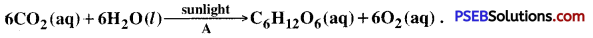What is A in above reaction.
Chlorophyll.

Question 35.
What is skeletal chemical equation?
If in a chemical reaction, number of atoms of each element on left hand side is not equal to number of atoms of each element on right hand side such a reaction is called skeletal chemical equation.

Question 36.
What are products?
In a chemical reaction new substances are formed which are known as products.

Question 37.
What are reactants?
Those substances which take part in a chemical reaction and undergo chemical changes are called reactants.

Question 38.
What is reduction reaction?
It is a reaction in which there is loss of oxygen or gain of hydrogen.

Question 39.
What is oxidation reaction?
It is reaction in which there is loss of hydrogen or gain of oxygen.

Question 40.
If in a reaction one reactant is reduced and other is oxidised, what is name of such a reaction?
Redox reaction.

Multiple Choice Questions:

Question 1.
The chemical reaction given below represents :
BaCl2(aq) + Na2SO4(aq) →
BaSO4(s) + 2 NaCl(aq)
(A) Decomposition reaction
(B) Displacement reaction
(C) Combination reaction
(D) Double diplacement reaction.
(D) Double displacement reaction

Question 2.
The chemical reaction in which heat energy is given out is called:
(A) Exothermic reaction
(B) Endothermic reaction
(C) Polymerisation reaction
(D) All.
(A) Exothermic reaction

Question 3.
During electrolysis, hydrogen and oxygen are produced in the ratio by volume :
(A) 2:1
(B) 1:1
(C) 2:2
(D) 4:1.
(A) 2: 1.

Question 4.
The chemical formula of rust is:
(A) Fe2O3
(B) FeCO3
(C) Fe2O3 .xH2O
(D) FeCO3 xH2O.
(C) Fe2O3 .xH2O

Question 5.
Which is an example of a decomposition reaction?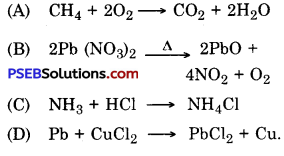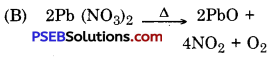Question 6.
Redox reaction involves:
(A) Oxidation
(B) Reduction
(C) Both oxidation and reduction
(D) None.
(C) Both oxidation and reduction

Question 7.
In a packet of chips oxygen is replaced by gas:
(A) CO2
(B) SO2
(C) N2
(D) O3.
(C) N2

Question 8.
Zn(s) + CuSO4(aq) → ZnSO4(aq) + Cu(s)
What type of chemical reaction is shown by the above equation?
(A) Combination reaction
(B) Dissociation reaction
(C) Displacement reaction
(D) Double displacement reaction.
(C) Displacement reaction.

Fill in the blanks:

Question 1.
Complete the following chemical equation.